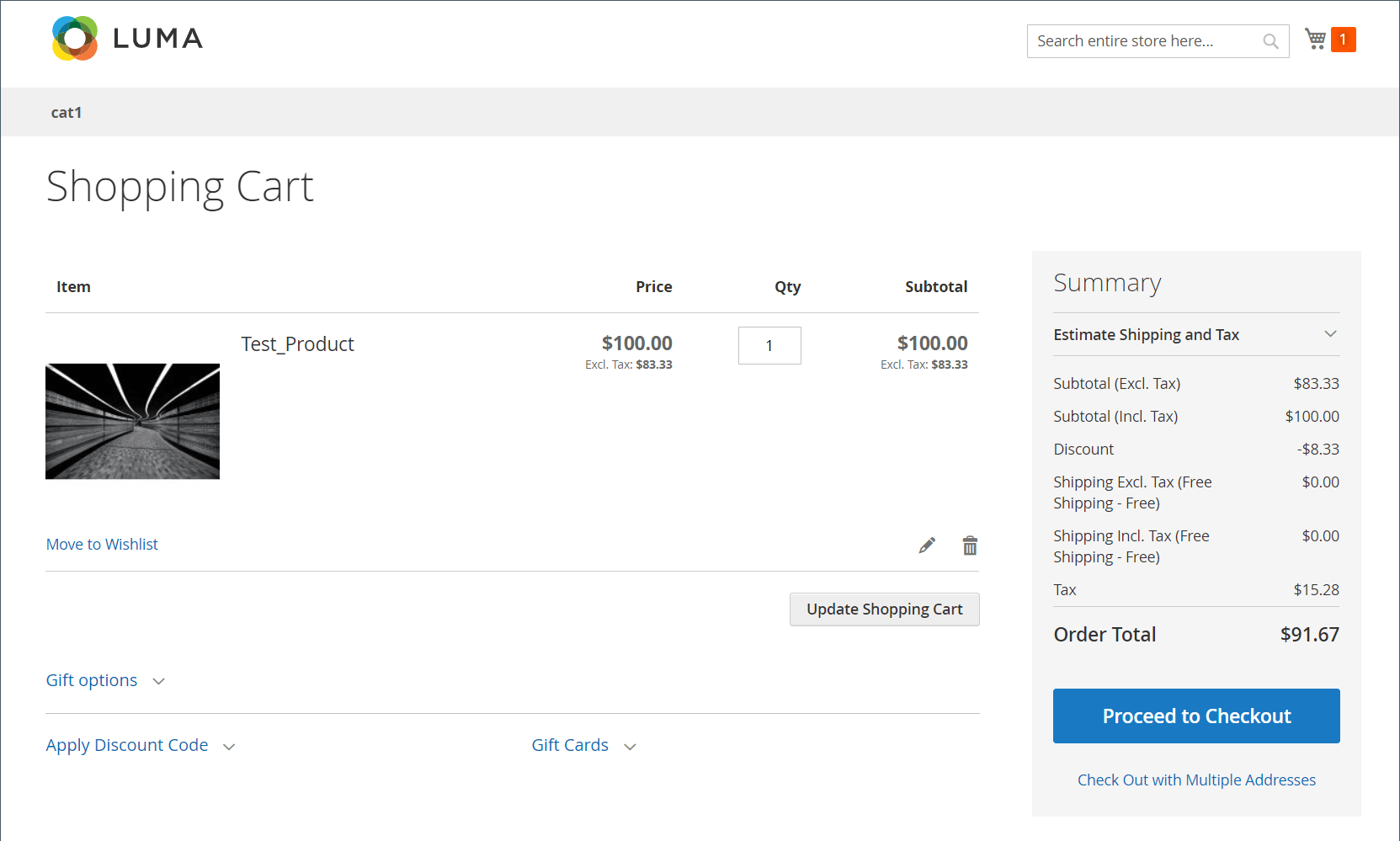# Hidden tax calculation

Hidden Tax is the amount of VAT that a discount amount has. It is non-zero when all of these conditions are true:

• Catalog prices include tax
• The VAT rate is not zero
• There is a discount present

When there is a discount that has tax embedded in it, Commerce computes a hidden tax that is added back for calculating the discounted price.

`discountedItemPrice = fullPriceWithoutTax - discountAmountOnFullPriceWithoutTax + vatAmountOnDiscountedPrice + hiddenTax`

## Example

1. Full price of item, with tax included: \$100
2. VAT at: 20%
3. Discount of 10% applied on item price-excluding taxes:

### Invalid Expected Result

• Item price after tax without discount=100 USD
• Item price before tax without discount=100/1.2=83.33 USD
• Discount=83.33 \ *0.1=8.33 USD
• Tax=(83.33-8.33) \ *0.2=15 USD (invalid)
• Order Total Excluding Tax=83.33-8.33=75 USD (invalid)
• Order Total Including Tax=75+15=90 USD (invalid)

### Valid Actual Result in Cart### Valid Calculations

1. Full price of the item without taxes is: \$100 / 1.2 = \$83.33

2. VAT amount on the full item price is: \$100 - \$83.33 = \$16.67

Can also be calculated as: \$100 \ * (1 - 1/1.2).

3. Discount of 10% on \$83.33 is: \$8.33 (when you don't discount tax)

4. Discounted price of item with tax is: \$100 - \$8.33 = \$91.67

NOTE

This equation illustrates the customer’s perception of how discounts are applied.

5. Discounted price of the item without taxes is: \$91.67 / 1.2 = \$76.39

6. VAT amount on the discounted price is: \$91.67 - \$76.39 = \$15.28 (valid)

Can also be calculated as: \$91.67 \ * (1 - 1/1.2).

7. Hidden tax or Discount Tax Compensation is the difference between the VAT amount of the full price versus discounted price: \$16.67 - \$15.28 = \$1.39

Another way to look at it: hidden tax is the VAT amount carried within the \$8.33 discount: \$8.33 * (1 - 1/1.2).

8. How the customer usually understands the discounted price (Order Total):

Full price of item including taxes less the discount amount: \$100 - \$8.33 = \$91.67

9. How Commerce calculates the discounted price (see earlier for formula):

\$83.33 - \$8.33 + 15.28 + 1.39 = \$91.67*